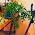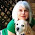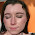### MATH 1280 Introduction to Statistics – Learning Journal Unit 4 – Part 1

Zebra, painted by Andy Warhol

We are in the middle of statistic course now. At this 4th week, I start with reading “learning guide unit 4” to get understand about what statistic topics will be learned.

Then, I write “discussion assignment unit 4.” After completion of discussion assignment, I post it to the forum. Read other peer’s posts, and give the comments.

Moreover, I study the topics of fourth week that are the histogram, the box plot, Median, first and third quartiles and the inter-quartile range, outliers, the mean (average), Skewness, the (sample) variance and standard deviation, and measuring the distance of a value from the mean.

I think have no problems to understand these topics. However, the assignment unit 4 is quite challenging to complete.

Tomato soup, painted by Andy Warhol

I completed the assignment with one question is quite doubting to answer. Then, I am writing journal about fourth week activities. After completing the journal, I post it for grading.

2. Vocabulary and R functions
a) What does the symbol x-bar represent?
x-bar represents sample mean

b) What does the Greek letter mu (μ) represent as it was used in this week's lessons?
mu (μ) represents population mean

c) What is the difference between x-bar and mu?
First, x-bar for sample, (μ) for population in regard to mean. Then, mean for population is a parameter, while mean for sample is a statistic.

# To be continued to part - 2

1.I like Andy Warhol.

1.Thank you to visit:
MATH 1280 Introduction to Statistics – Learning Journal Unit 4 – Part 1
-
Zebra, painted by Andy Warhol

2.Picture 1 amazing 😊

1.Thank you to visit:
MATH 1280 Introduction to Statistics – Learning Journal Unit 4 – Part 1
-
We are in the middle of statistic course now. At this 4th week, I start with reading “learning guide unit 4” to get understand about what statistic topics will be learned.

3.I love Andy Warhol, his art is beautiful.

1.Thank you to visit:
MATH 1280 Introduction to Statistics – Learning Journal Unit 4 – Part 1
-
Then, I write “discussion assignment unit 4.” After completion of discussion assignment, I post it to the forum. Read other peer’s posts, and give the comments.

4.I always loved the paintings of Andy Warhol. These two paintings are so different
and so amazing!!!

1.I see

Thank you to visit:
MATH 1280 Introduction to Statistics – Learning Journal Unit 4 – Part 1
-
Moreover, I study the topics of fourth week that are the histogram, the box plot, Median, first and third quartiles and the inter-quartile range, outliers, the mean (average), Skewness, the (sample) variance and standard deviation, and measuring the distance of a value from the mean.

5.Love the zebra☺

1.Thank you to visit:
MATH 1280 Introduction to Statistics – Learning Journal Unit 4 – Part 1
-
I think have no problems to understand these topics. However, the assignment unit 4 is quite challenging to complete.

6.Oh evry interesting darling
xx

1.Thank you to visit:
MATH 1280 Introduction to Statistics – Learning Journal Unit 4 – Part 1
-
I completed the assignment with one question is quite doubting to answer. Then, I am writing journal about fourth week activities. After completing the journal, I post it for grading.

7.how cool is this zebra ☺
best regards
Lili

1.Thank you to visit:
MATH 1280 Introduction to Statistics – Learning Journal Unit 4 – Part 1
-
2. Vocabulary and R functions
a) What does the symbol x-bar represent?
x-bar represents sample mean

8.I love the pictures from Andy Warhol. Have a nice november.

1.Thank you to visit:
MATH 1280 Introduction to Statistics – Learning Journal Unit 4 – Part 1
-
b) What does the Greek letter mu (μ) represent as it was used in this week's lessons?